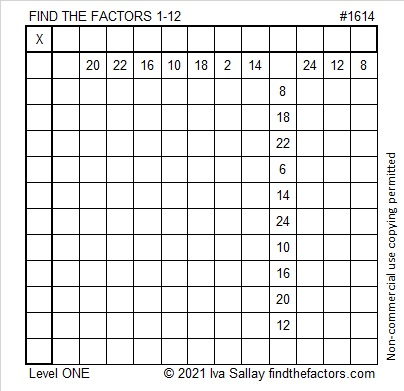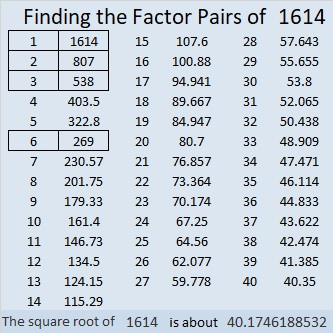# 1614 and Level 1

Contents

### Today’s Puzzle:

Write the numbers from 1 to 12 in both the first column and the top row so that the puzzle functions like a multiplication table.### Factors of 1614:

1614 is even so it’s divisible by 2.

Here’s the weird thought process I used to determine its divisibility by 3:
161514 is made from three consecutive numbers, so it’s divisible by 3. We know that 15 is divisible by 3, so we can remove it. That means 1614 is divisible by 3.

Since 1614 is divisible by both 2 and 3, it is divisible by 6.

• 1614 is a composite number.
• Prime factorization: 1614 = 2 × 3 × 269.
• 1614 has no exponents greater than 1 in its prime factorization, so √1614 cannot be simplified.
• The exponents in the prime factorization are 1, 1, and 1. Adding one to each exponent and multiplying we get (1 + 1)(1 + 1)(1 + 1) = 2 × 2 × 2 = 8. Therefore 1614 has exactly 8 factors.
• The factors of 1614 are outlined with their factor pair partners in the graphic below.### More about the Number 1614:

1614 is the hypotenuse of a Pythagorean triple:
414-1560-1614, which is 6 times (69-260-269).

1614 is also a leg in two Pythagorean triples because
2(807)(1) = 1614, and
2(269)(3) = 1614.

You can calculate those Pythagorean triples by letting a be the first number in parenthesis for each of those equations, and b be the second number in parenthesis. Then substitute those values in the three expressions below, and you will have some Pythagorean triples!
2(a)(b), a² – b², a² + b².

This site uses Akismet to reduce spam. Learn how your comment data is processed.